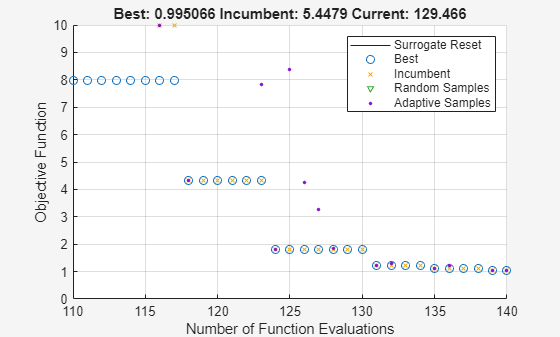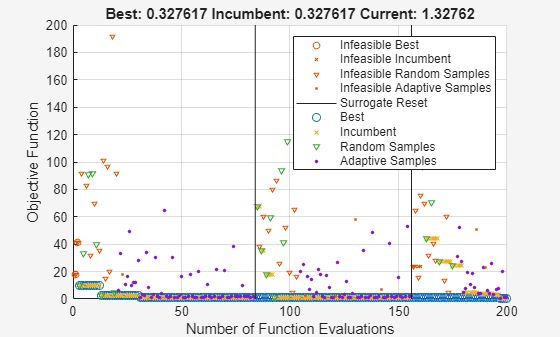# Interpret `surrogateoptplot`

The `surrogateoptplot` plot function provides a good deal of information about the surrogate optimization steps.

### Minimize Bounded Function

For example, consider the plot of the steps `surrogateopt` takes on the test function `rastriginsfcn`, which is available when you run this example. This function has a global minimum value of 0 at the point [0,0].

Create a surface plot of `rastriginsfcn`.

`ezsurf(@(x,y)rastriginsfcn([x(:),y(:)]));`### Plot Minimization Process

By giving asymmetric bounds, you encourage `surrogateopt` to search away from the global minimum. Set asymmetric bounds of `[-3,-3]` and `[9,10]`. Set options to use the `surrogateoptplot` plot function, and then call `surrogateopt`.

```lb = [-3,-3]; ub = [9,10]; options = optimoptions('surrogateopt','PlotFcn','surrogateoptplot'); rng(100) [x,fval] = surrogateopt(@rastriginsfcn,lb,ub,options);``````surrogateopt stopped because it exceeded the function evaluation limit set by 'options.MaxFunctionEvaluations'. ```

### Interpret Plot

Begin interpreting the plot from its left side. For details of the algorithm steps, see Surrogate Optimization Algorithm.

• The first points are colored triangles, indicating quasirandom samples of the function within the problem bounds. These points, labeled "Random Samples" in the legend, come from the Construct Surrogate phase.

• Next are dots indicating the adaptive points, the points created in the Search for Minimum phase. These points are labeled "Adaptive Samples" in the legend.

• The circles (overlapping to look like a thick line) represent the best (lowest) objective function value found. These points are labeled "Best" in the legend. Shortly after evaluation number 30, `surrogateopt` is stuck in a local minimum with an objective function value near 8. Zoom in to see this behavior more clearly.

```xlim([20 100]) ylim([0 15])```• Before evaluation number 120, a vertical line indicates a surrogate reset. At this point, the algorithm returns to the Construct Surrogate phase.

• The colored x points represent the incumbent, which are the best points found since the previous surrogate reset.

• Near evaluation number 150, the incumbent improves on the previous best point by attaining a value of about 4. Zoom in to see this behavior more clearly.

```xlim([140 200]) ylim([0 6])```• The solver has another surrogate reset after evaluation 190.

• The optimization halts at evaluation number 200 because it is the default function evaluation limit for a 2-D problem.

### Problem with Nonlinear and Integer Constraints

The `surrogateoptplot` display changes when you have nonlinear constraints. Impose the constraint that `x(1)` is integer-valued, and the nonlinear constraint that ${x}_{2}\ge {x}_{1}^{2}-2$. For the function that implements this constraint, see `rasfcn` at the end of this example.

`fun = @rasfcn;`

Set integer constraints by setting `intcon` = 1, and run the minimization.

```intcon = 1; [x,fval] = surrogateopt(fun,lb,ub,intcon,options);``````surrogateopt stopped because it exceeded the function evaluation limit set by 'options.MaxFunctionEvaluations'. ```

The plot now shows colored markers where `surrogateopt` evaluates infeasible points. The final point is close to the true minimum point of [0,0].

`disp(x)`
``` 1.0e-04 * 0 0.6798 ```

The integer constraint likely helps `surrogateopt` find the true minimum by reducing the search space.

```function F = rasfcn(x) F.Fval = rastriginsfcn(x); F.Ineq = x(1)^2 - 2 - x(2); end```